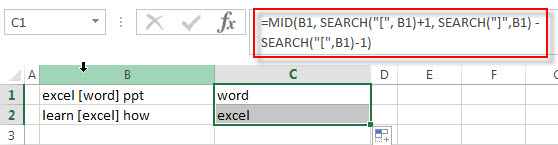# How to Extract Text between Brackets

We talked that how to extract data between parentheses in the previous post, and this post will guide you how to create similar formula to extract text between brackets in a cell.

## Extract text between brackets

If you want to extract text between brackets in a cell, you need to create a formula based on the SEARCH function and the MID function.

The MID function will extract a specific number of characters at a specific starting position. You need to know how to get the starting position of text between brackets in a test string, you can use the SEARCH function to get the position of the first left/right bracket characters.

Next, you can get the starting position of the text that you want to extract and the length of the text. So we can write down the following excel formula:

=MID(B1, SEARCH(“[“, B1)+1, SEARCH(“]”,B1) – SEARCH(“[“,B1)-1)For more detailed information about this formula, you can continue to read the below post:  how to extract text between parentheses in excel.

### Related Formulas

• Extract Text between Parentheses
If you want to extract text between parentheses in a cell, then you can use the search function within the MID function to create a new excel formula…
• Check If Cell Contains All Values from Range
If you want to check if a cell contains all values in a list, you can use a combination of the SEARCH function, the SUMPRODUCT function, the ISNUMBER function and COUNTA function…

### Related Functions

• Excel SEARCH function
The Excel SEARCH function returns the number of the starting location of a substring in a text string.The syntax of the SEARCH function is as below:= SEARCH  (find_text, within_text,[start_num])…
• Excel MID function
The Excel MID function returns a substring from a text string at the position that you specify.The syntax of the MID function is as below:= MID (text, start_num, num_chars)…

Sidebar